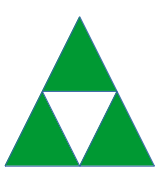# TCS Placement Paper | MCQ 7

• Difficulty Level : Medium
• Last Updated : 21 May, 2019

This is a TCS model placement paper for aptitude preparation. This placement paper will cover aptitude questions that are asked in TCS recruitment drives and also strictly follows the pattern of questions asked in TCS interviews. It is recommended to solve each one of the following questions to increase your chances of clearing the TCS interview.1. The sticks of the same length are used to form a triangle as shown below. If 87 such sticks are used then how many triangles can be formed?a) 42
b) 43
c) 44
d) 45

`Answer: b) 43`

Solution:
As we can see the first triangle can be formed using 3 sticks. So we have 87 – 3 = 84 sticks left.
So every next triangle can be formed using 2 sticks.
So we have 84/2 = 42 triangles and 43 triangles in all.

2. Find the next number in the series of 3, 12, 7, 26, 15, ?
a) 54
b) 55
c) 64
d) 74

`Answer: a) 54`

Solution:
3 * 2 + 1 = 7
12 * 2 + 2 = 26
7 * 2 + 1 = 15
26 * 2 + 2 = 54

3. There is a toy gun that made 10 musical sounds. It makes 2 musical sounds after being defective. What is the probability that same musical sound would be produced 5 times consecutively?
a) 1/16
b) 1/32
c) 1/48
d) 1/2

`Answer: b) 1/32`

Solution:
The probability of making the same sound every time = 1/2,

4. In how many possible ways you can write 3240 as a product of 3 positive integers?
a) 320
b) 420
c) 350
d) 450

`Answer: d) 450 ways`

Solution:
First let’s prime factorize the number 3240 =Let the three positive numbers be x, y and z
We have to distribute three 2’s to x, y and z ways in (3+3-1)C(3-1) = 5C2 ways = 10 ways
We have to distribute four 3’s to x, y, z in (3+4-1)C(3-1) = 6C2 ways
We have to distribute one 5 to x, y, z in 3 ways.
The total number of ways = 10×15×3=450 ways.

5. The marked price of a shirt was 40% less than the suggested retail price. Ram purchased the coat for half of the marked price at the 15th-anniversary sale. What per cent less than the suggested retail price did Ram pay?
a) 70%
b) 20%
c) 60%
d) 30%

`Answer: a) 70%`

Solution:
Let the retail price of the shirt be Rs. 100
So according to the question, the market price will be = 100*0.6 = 60
Purchased price of Ram = 60/2 = 30
which is 70% less than retail price.

6. HCF of 2472, 1284 and a 3rd number, is 12. If their LCM is 8*9*5*103*107, then what is the number?
a) 2^2*3^2*7^1
b) 2^2*3^2*5^1
c) 2^2*3^2*8103
d) None of the above.

`Answer: b) 2^2×3^2×5^1`

Solution:
2472 =1284 =HCF =LCM =HCF of the number is the highest number which divides all the numbers. So N should be a multiple of 22×3
LCM is the largest number that is divided by the given numbers. As LCM contains 32×5 these two are from N.
So N = [Tex]\$2^2×3^2×5^1\$[Tex]

7. An old man takes 30 minutes and a young man takes 20 minutes to walk from apartment to office. If one day the old man started at 10.00 AM and the young man at 10:05 AM from the apartment to office, when will they meet?
a) 10:00
b) 10:15
c) 10.30
d) 10:45

`Answer: b) 10:15`

Solution:
Let the distance of the apartment from the office be 12 km
So the speed of the old man = 12 / (1/2) hr = 24 km/hr
The young man speed = 12 / (1/3) hr = 36 km/hr
Since the old man started 5 minutes earlier, he covers 24 × (5/60) = 2 km in 5 minutes.
Now the time taken to the young man to meets him = 2/(36-24) * 60 = 10 minutes
So the time of meet = 10:05 + 10 = 10 hr 15 min or 10:15

8. In the range of 112 to 375, how many 2’s are there?
a) 312
b) 156
c) 159
d) 160

`Answer: b) 156`

Solution:
The total number of 2’s in the units place = (122, 132, 142 … 192), (201, 212, 222, … 292), (302, 312, … 372) = 8 + 10 + 8 = 26 2’s
The total number of 2’s in tenth’s place = (120, 121, 122, …, 129) + (220, 221, …, 229) + (320, 321, …, 329) = 30
The total number of 2’s in hundred’s place = (200, 201, … 299) = 100.
So the total number of 2’s between 112 and 375 = 26 + 30 + 100 = 156

9. Ram walks 36 km partly at a speed of 4 km/hr and partly at 3 km/hr. If he had walked at a speed of 3km/hr when he had walked at 4 and 4 km/hr when he had walked at 3 he would have walked only 34 km. The time (in hours) spent by Ram in walking was
a) 10
b) 5
c) 12
d) 8

`Answer: a) 10`

Solution:
Let Ram walk ‘x’ hrs at 4 km/hr, and ‘y’ hrs at 3 km/hr.
Given,
4x + 3y = 36
3x + 4y = 34
Solving these two equations we get x + y = 10

10. What will be the 55th word in the arrangement of the letters of the word PERFECT?
a) CEPFRET
b) CEPFERT
c) CEPERFT
d) CEPRFET

`Answer: b) CEPFERT`

Solution:
Let’s arrange the word PERFECT in dictionary order = CEEFPRT
Here,
CEE(4!)=24
CEF(4!)=24
CEPF(3!)=6
So the 55th word is CEPFERT.

My Personal Notes arrow_drop_up
Recommended Articles
Page :